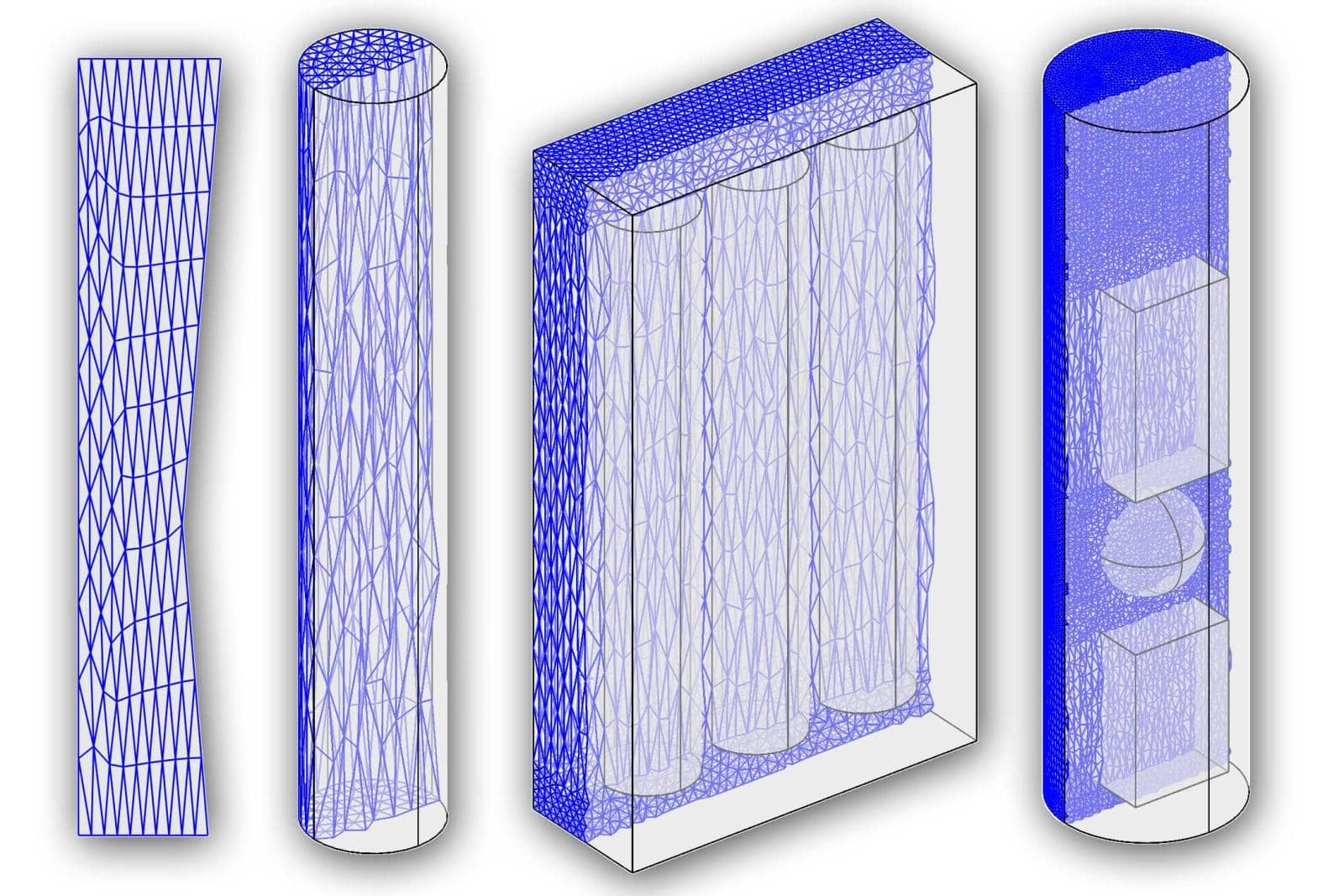# Grid Generation with ScalingFor models and geometries where either one axis direction dominates, such as for long or tall geometry objects, or simulations where the solution is expected to not to vary in certain directions, such as some flow situations, it can be very beneficial to use anisotropic grids. By using elongated mesh elements the total mesh size can be reduced resulting in significantly faster simulations (especially for full 3D simulations).

Unfortunately, most automatic mesh generation algorithms try to avoid anisotropic mesh elements, so if the geometry allows for it, the best way to generating such anisotropic meshes is by manually generating structured meshes. If that isn’t possible, another approach introduced here is to first scale the relevant parts of the geometry, mesh the scaled geometry, and the re-scale the mesh back to fit the original size. The grid generation function linked below allows for anisotropic meshes to be generated using this approach.

MATLAB Function for Automatic Grid Generation with ScalingFor example, the following command generates a mesh for a polygon with elements stretched by a factor of 5 in the y-direction (left image).

``````grid = gridgen_scale( gobj_polygon([0 0;1 0;0.8 2;1.1 5; 0 5]), 'axis', 2, 's_scal', 1/5 );
plotgrid( grid )
``````

And as a more complex example, here two blocks embedded in an outer cylinder are scaled by a factor of 2 and 4, respectively (right image).

``````s1 = gobj_sphere();
b1 = gobj_block(-1,1,-1,1,1.5,4.5);
b2 = gobj_block(-1,1,-1,1,-3.5,-1.5,'B2');
c1 = gobj_cylinder([0,0,-4],1.75,9,3);
geom.objects = {s1, b1, b2, c1};

grid = gridgen_scale( geom, 'axis', 3, 'p_scal', [-2.5, 3], 's_scal', [1/2, 1/4] );

plotgrid( grid, 'selcells', 'y>0' )
plotsubd( grid, 'linewidth', 1, 'labels', 'off' )
plotedg( grid, 'colors', {'k'}, 'labels', 'off', 'linewidth', 1 );
view(3)
``````